ECE 476 Final Project

Retractable Alarm Clock (RAC)

Colleen Carey, Vincent Zou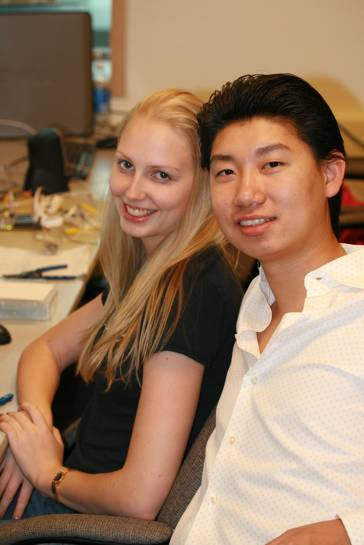# Index:

## 1     Project Concept

1.1      Motivation

1.2      Introduction

3  Hardware Components

3.1      motors

3.2      LEDs

3.3      Switches

3.4      Speakers

3.6      optoisolator

4  Software Design

4.1      DDS

4.2      Timing

5  Designs and Testing

5.1      Design Flow

5.2      Challenges

6  Packaging

6.2      Motor box

6.3      Alarm case

7  Cost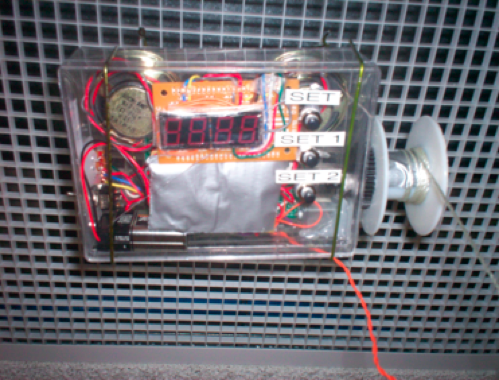### 1.1  Motivation:

Alarm clocks are essential in almost everyone’s daily life.  For most of us, we start our day to the sweet noise of our alarms.  While some people wake up instantaneously to the first chirp of an alarm, some struggle everyday to get out of the bed. The Retractable Alarm Clock is the next generation alarm clock that combines both active alarm feature and penalty system that trains the users to wake up to the alarm over time. The clock in this projects consists of a head unit that is to be hanged on the ceiling above the bed and has a retractable snooze button which hangs to reachable height above the bed. When the user snooze the alarm, the snooze button moves up, promoting the user to physically get up and to snooze the next time.

### 1.2  Introduction:

By using an ATMega32 chip, the alarm clock can generate different alarm sounds. The time is displayed on LED lights.  A customized snooze button is made to accommodate the movement of the retractable cord powered by a small dc motor. The alarm gradually increases the loudness to wake up sleepers gently. To prevent the sleeper from falling asleep to the monotonic sound of alarms, our alarm varies the frequency every 12 chirps.

## 2     High Level Design:

### 2.1  Hardware Block Diagram Overview:

The following block diagram describes our basic hardware layout: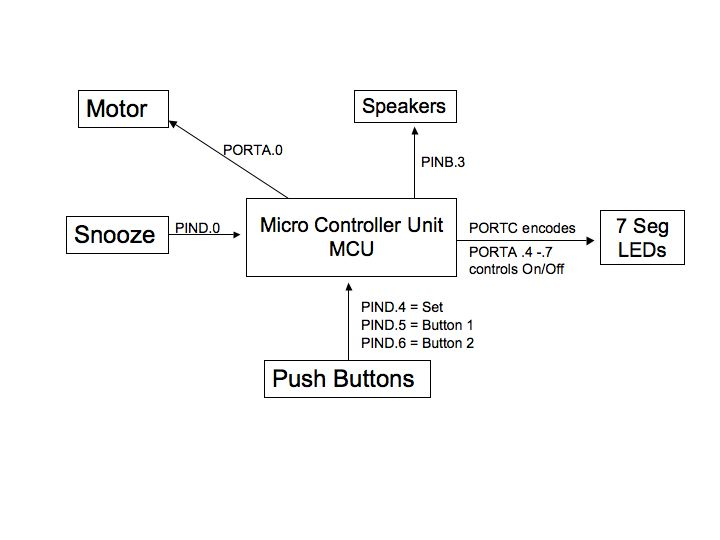### 2.2  Software State Diagram:

The following block diagram describes our basic software layout:

## 3.    Hardware Components:

### 3.1      Motors: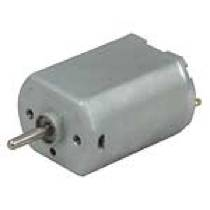For this project, we tried five different motors.  The fan motor used in Lab 5 does not provide the required torque.  The stepper motors that were available in the lab require too many control lines.  Because we have limited ports off the ATMEGA32, we would have to have used a multiplexer.  Also, the only function that we require the motor to do is to spin in one direction and thus using a stepper motors seems like a waste if its potential.  We then found a broken electrical toothbrush and took the motor out of it.  The electrical toothbrush runs on a 1.5V AA battery. The entire circuit that we build is based 5V so to use the motor from the toothbrush we would have had to step down the voltage before it entered the motor.  However, by doing so, we also decrease the voltage going in to the 4N35 chip that is used to control the motor.  The voltage drop across the 4N35 is about 0.7 V, when running on a 5-12V, this drop seems ignorable, but if a 1.5V is applied, the drop is almost half of the voltage and thus will not power the motor. We then ordered a 9V DC motor from All Electronics, however we did not use it because it draws 1.35 amps, and is not suitable for our circuit.  We also acquired a fourth motor from another group that was not using it.  The motor was not new and had several connection issues originally.  Once we got it working we found that it heats up the BUZ73 very fast and drains the battery dry in just a few minutes.

So after exploring all options, the motor we finally decided to use is a +5V DC motor that was ordered from Jameco (PIN231802) that came in later than we expected.  The motor requires around 0.15 amps to operate and has a reasonable battery life. This motor is brand new and has no connection problems.  The motor still heats up the 4N35 chip but it is not significant because we only run the motor for less than one second at a time, so the heating problem can be ignored.

### 3.2      LEDs: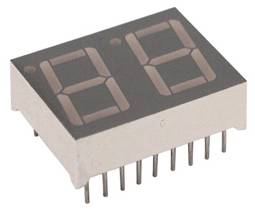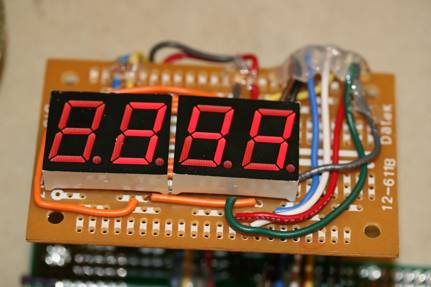Since it is an alarm clock, the user needs to be able to read the time at night from a reasonable distance. A lighted LCD is too hard to read even though it would be much easier to integrate. We used four seven segment displays. Under different settings, the display will show the Current time, Alarm set time, Current Date, and Current Day of the Week. Once the set button is pressed, the display settings change in order to show the current setting on the LEDs.  This means that if the set button is pressed once, the LEDs will show the month and date.  Pressing the set1 and set2 buttons will change the month and date, respectively.  Once the set button is pressed again, the current time is displayed.  Pressing the set1 and set2 buttons will change the hour and minute, respectively.  One more press of the set button will change the LEDs to display the current day of the week.  Pressing the set1 will increase the day and set2 will decrease the day.  Pressing set a final time will return the display to the current time after all settings are made.

To power all four LEDs at all times requires a considerable amount of current and drains the battery.  Also, if we wanted to code each 7-segment display individually, 36 control lines and pins would be required.  Due to the above two limitation, we could not control individual LEDs separately. To solve this problem, we wired all the LEDs in parallel to the same output pins.   This method required each LED display to have its power controlled separately.  The total configuration required 12 pins.  By precisely timing the refresh rate and on and off of the individual LEDs, we can flash the 4 LEDs in sequence and change the display between each flash. Human eyes can’t detect the change as long as it flashes at a reasonable rate. We light each LED for 5ms before switching to the next LED.  To the viewer, the flash is not detectable.

Speakers and Motors drain a significant amount of current from the battery; to run them all at the same time results in overheating Buz73 and significant dimming of LEDs. To solve this problem, we cycle through the 3 components the same way we flash the LEDs.  When the Alarm goes off, LED displays flash every 5 ms and cycle 40 times before the signal is sent to create a beep at the speakers.  Once the signal is sent the LEDs turn off until the speakers are silent.  Also, when the snooze button is pressed, the motor turns on and both the beeping and LEDs turn off.

### 3.3      Switches: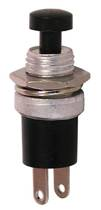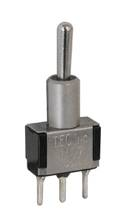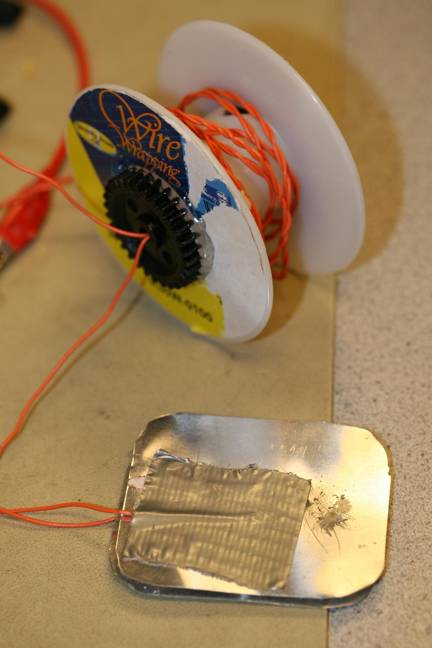We found several very basic switches from the box of extra/used supplies.  We also bought 3 pushbuttons from All Electronics.  Some of the push buttons are defaulting high and others are defaulting low.  For the snooze button, we need a push button large enough to be pressed easily.  Vincent made a default low push pad with two metal plates.  All of the push buttons are not factory debounced, so we debounced the set button, which determines which setting state the clock is in.  For the set1 and set2 buttons, we did not debounce them.  Instead we check them every 350ms so that if you hold them, a press will be seen every 350ms and the numbers will change.  This is beneficial to the user as it makes setting the clock easier than if each change required a button press.   All push buttons are connected to a pull down resistor to ground to ensure a clean signal with no flouting charges.

### 3.4      Speakers: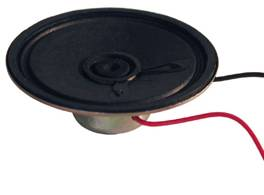The Alarm clock has two speakers so it looks symmetrical.  Only one speaker is need to produce an audible sound but two sounds better. We use two 8 Ohm, 0.25W, and 21/4-inch speakers from AllElectronics.com.  Unlike the speakers from the TV, those we have do not have built in amplifiers. The output from PINB.3 is too weak to power the two speakers so we built an emitter follower to boost the output of the speakers. Because of the extra circuitry that we added, some noise is produced. Since we only need the sound to be a sign wave of some kind, the quality of the sound it produces is very acceptable.

### 3.5  Emitter Follower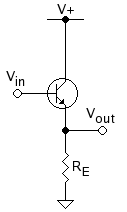# Voltage Gain: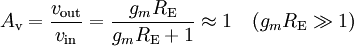Current Gain: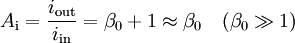Input resistance: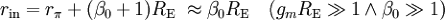# Output resistance: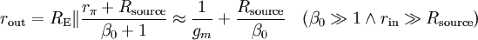gm is the transconductance in siemens, calculated by , where: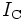is the quiescent collector current (also called the collector bias or DC collector current)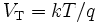is the thermal voltage, calculated from Boltzmann’s constant, the charge on an electron, and the transistor temperature in kelvins. At room temperature this is about 25 mV.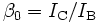is the current gain at low frequencies (commonly called hFE). This is a parameter specific to each transistor, and can be found on a datasheet.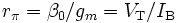## Rsource is the thevenin equivalent source resistance.

To maximize the gain, we used a 10K for RE. The Speaker is turned on monetarily and does not over heat.

### 3.6  Optoisolator: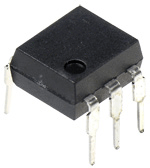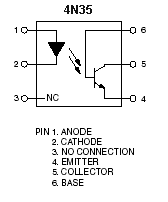The Motor is separated from the MCU through an Optoisolator (4N35). The Motor draws a significant amount of current and it is most safe if runs separately.

## 4     Software Design

### 4.1  Alarm/DDS:

Once triggered, the alarm can be only turned off by pressing the snooze button or shutting off the RAC. If untreated, the alarm will only sound for a max of 10 mins to prevent battery waste.

## The alarm sound consists of:

15 sec of regular alarm,

Pronounced Day of the week and Date. (i.e. today is Friday, April 27th 2007)

15 sec of a second alarm sound with higher frequency

15 sec of the third alarm sound with an even higher frequency

15 sec of the fourth alarm sound with very high frequency

## Repeat the process for 5 mins

After much thoughts and consideration, we found that the most annoying and effective alarm sound to be the cricket calls from Lab two. So the DDS codes are based on the codes and programs that we used in the Cricket call synthesizer in laboratory assignment 2.  The PWM signal will be sine wave bursts generated using amplitude modulated Direct Digital Synthesis (DDS) technique. This is the most complicated part of this lab. A cricket call may be very complex, but we will limit ourselves to the following form.

1. A call is made up by repeating indefinitely a chirp and a silent period. If the duration of the silence is zero, the call is referred to as a trill.

2. A chirp is made up of a certain number of syllables with specific duration and repetition interval.

3.  A syllable is made up of a sine wave burst at a certain frequency. The burst starts at low amplitude, which increases quickly to a maximum, sustains the maximum amplitude until just before the end, and then decreases amplitude to zero.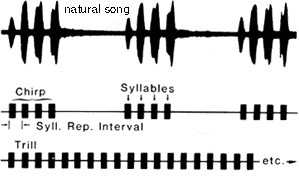to create a simulated cricket song, we use sine waves. To generate a sine wave we use the function sineTable:

for (i=0; i<256; i++)

begin

sineTable[i] = (char)(127.0 * sin(6.283*((float)i)/256.0)) ;

end

Now to make the cricket call smooth, we used an amplitude modulation to envelope the sine wave by creating a 4 ms gradual rising slope and 1 ms drop at the end of the chirp.  The original code will only generate continuous chirps and needed to be modified to generate silence periods and repeat intervals.  To do this we used while loop and for loops.

1. We first created a timer that counts every ms and a syllable counter that counts every time a syllable has been fully produced.

2. We then enter a while (1) loop to keep the signal generation continuous.

3. Then we check if time has exceeded the repeat interval and if the counter has completed all the syllables. If neither condition has been satisfied we execute the for loop, ram up 4 ms, generate full signal for a specified length and ram down in 4 ms. This will generate one more syllable.

4. We keep running the for loop until all syllables have been generated.

5. Then we silent the song and keep the time running for a specified period of time.  (chirp_silence -syllables*repeat_int will give the silent perios).

### 4.2  Timing

Because we use timer0 to control our clock and the dds portion, we have the prescalar set to 0, so the clock is free running.  We record 1ms every 62 counts of the clock.  We then increase the value of minutes every 60,000ms.  From the value of minute we calculate hour, day, month, and date.   This value will be accurate to the accuracy of the crystal, which is 1 part per million.

## 5     Designs and Testing

### 5.1  Design Flow

Vincent was responsible mainly for hardware and Colleen did most of the coding. We designed and tested each component separately and put all of everything together at the end.  The Motor took a fair amount of time to set up. See the Motor section. The LEDs also gave us some problems because we had to figure out the exact flash rate for it to look like all four LEDs were continuously on.  The circuit for the speakers was fairly simple but we tried to use an amplifier to boost the output. At the end we decided to use Emitter Follower. Combining the motor, speakers, and the LEDs consumed a lot of our time because the 9V battery is very weak to power all of them at the same time. Because the alarm clock requires all three functions at the same time, we have to switch between the three at a fast enough rate so the user does not notice.

### 5.2  Challenges

#### 1.    Motor strength:

Originally what we wanted to have the entire alarm unit hang from the Ceiling and have it all move up as snooze button is pressed. The entire unit weighs too much to lift in this way.  This requires too much motor strength and too much power. So to solve this problem we have a single retractable snooze button that will be pulled up once the user snoozes the alarm.  We build a great box to have the gear ratio 4:1 at this rate the speed is a little slow but we have enough power to overcome the weight of the button.

#### 2.    Speaker Loudness:

Certain chirp setting generates a very low sound. With syllable set to one or five, the loudness decreases to one fifth of those other setting for no obvious reasons. Instead of treating this as a challenge, we use this towards our benefit. Sleepers are usually annoyed by sharp alarm sounds and studies shows that gradual increase of loudness is more efficient to wake them up.   So we set the first chirping sequence with syllable one and create low amplitude and then gradually increasing it. We alternated the frequency of the alarm clock so user does not get use to the monotonic sound and interpret it as a constant white noise and fall asleep to it.

#### 3.    Voice generation

In our original design we have a voice generation function that will announce the Date and Day. We collected all the voice data and tried them out in a separate voice code. Everything works well separately but when we integrate them in the alarm code, things got weird and nothing works. We ran out of time at the end to fix this problem.

## 6     Packaging

### 6.1  Retractable Cord

We made the retractable cord with metal plates with a wire attached to each of them.   One plate is set high and when the two are in contact, the second plate will be set high.

### 6.2  Alarm Case

The motor is not powerful enough directly pull up the snooze button. So we step down the gear ration to 4:1. So 4 full turn of the motor produces 1 full turn of the spindle.

### 6.3  Alarm case

The Alarm case is made of clear plastic.  We found the box in the wastebasket.  In order to add all of our hardware we had to create several holes for the buttons, switches, and speakers.

## 7     Cost

### Cost:

 Item Unit Price (\$) Unit Total ATMEGA 32 Chip 8.00 1 8.00 Custom PC Board 5.00 1 5.00 5V DC motor 3.24 1 3.24 Small Solder Board 1.00 1 1.00 Speakers 1.50 2 3.00 9V Battery 2.00 1 2.00 Push buttons 0.05 4 0.20 7 seg LEDs free 4 0.00 Gears 0.2 2 0.4 Total 22.64

#### 8 Ethics

Our Project is an extension and collection of many labs that were completed during the semester. Many circuit are similar to those that we built during the class. However, everything has to be modified and customized to work with our design requirement.  Professor Bruce Land provided many useful codes that proved to be very helpful.  Also, many of the diagrams used in this report are on the course website, from different labs. Much credit has go to our beloved TAs for their consistent help.  Most of our parts and components are gathered from the wastebasket and are not counted toward our cost table. Before the project, we researched the concept of motorized alarm clock and found several different designs. None of the designs that we found have any data sheets or instructions on how to build them. So even that the concept of a motorized alarm is not groundbreaking, building one still requires considerate amount of high-level and detailed design and testing.  All the actions that we took designing this project are consistent with the IEEE Code of Ethics.

##### 9     Conclusion

The project lasted for four weeks. In these four weeks, we explored many topics in Microcontrollers ranging from programming and software to amplification, power regulation, and optoisolators. Most of the functions are completed according to our timetable.  It is very satisfying to design a product and build every part of it and to see everything work together.   We had many trials along the way, so this project has helped us to really understand much of what we have learned in all of our courses at Cornell.   In order to configure and debug our code and hardware we had to use knowledge from almost every course we have taken up to this point.  As graduating seniors, this project has been a great end to our Cornell education, as we truly had to apply all of our knowledge.

10        Commented Code:

//******************************************//

//                                           //

//   Final Project                            //

//   Colleen Carey & Vincent Zou             //

//   Wednesday 4:30-7:30                     //

//   final_proj.c                            //

//                                          //

//******************************************//

#include <Mega32.h>

#include <math.h>                               // for sine

//timeout values for each task

#define t1 75                                                                                     // time for debounce

#define t3 20                                   // time for button check

#define t4 25                                      // time for display

#define t5 350

#define begin {

#define end }

#define minute 60000                                                                   // one minute

#define countMS 62                                 // ticks/mSec

//define setting states

#define set_normal 1

#define set_month_date 2

#define set_hour_min 3

#define set_day 4

#define set_alarm 5

//define debounce states

#define no_push  1

#define maybe_push  2

#define push  3

#define maybe_nopush 4

//the task subroutines

void debounce(void);                                                              // debounces

void clk_display (void);                        // controls clock settings

void update_time(void);                                                                // update time

void initialize(void);                                                                 // initialize everything

void snooze(void);

void motor(void);                                                                                 // runs motor

void decode(void);

void alarm_sound(void);

unsigned int push_flag;

unsigned int time, time1, time2, time3, time4, time5;    // timeout counters

unsigned char set_state, push_state;     // switch statements

unsigned int numb_set_pushed;

unsigned int alarm, alarm_flag, alarm_count, snooze_count, alarmSet;

unsigned int c, i, MotorOn;

//define dds variables

unsigned long accumulator @0x2f0;

unsigned char highbyte @0x2f3;                                          // the HIGH byte of the accumulator variable

unsigned long increment;

char sineTable @0x300;                                                       // need loc to avoid glitch

char amp, count;

unsigned int chirp_silence;                                                       // chirp+silence time

unsigned int syllables;                                                                // number of syllables

unsigned int syllable_time;                                                       // syllable duration

unsigned int repeat_int;                                                          // repeat interval

unsigned int burst_freq;                                                          // burst frequency

unsigned int counter;                                                                       // syllable counter

int display_1, display_2, hour, day, month, date, year, alarm_hour, alarm_min, snooze_int;                    // system time variables

int led1, led2, led3, led4, chirp_count, snooze_flag, alarm_set_mode;

int Min;

//**********************************************************

interrupt [TIM0_OVF] void sgen(void)

begin

//the DDR code and scaling

accumulator = accumulator + increment ;

OCR0 = 128 + sineTable[highbyte] >> amp ;

//generate rising amplitude

// 62 counts is about 1 mSec

count--;

if (0 == count )

begin

count = countMS;

time++;    //in mSec

if (time1 > 0)  --time1;                                        //timer 1 for debounce

if (time2 > 0)  --time2;                                        //timer 2 to count 1 minute

if (time3 > 0)  --time3;                                        //timer 3 for updating

if (time4 > 0)  --time4;                                     //timer 4 for clock update

if (time5 > 0)  --time5;                                     //timer 5 for push buttons

if (c > 0) --c;                                                                  //timer for motor

end

end     // end interrupt

//**********************************************************

// main

//Entry point and task scheduler loop

void main(void)

begin

initialize();

//main task scheduler loop

while(1)

begin

//1ms clock

if (time1 == 0)

begin

debounce();                                                                             // debounce runs every 50ms

end

if (time2 == 0)

begin

update_time();                                                               // system time updates time every min

end

if (time3 == 0)

begin

time3 = t3;

if (~PIND.6 == 0)                                                       // check if snooze button is pressed

begin

snooze_flag = 1;

snooze();

end

if (~PIND.0 == 0)                                       // check if the alarm is on

begin

alarmSet = 1;

end

else if (~PIND.2 == 0)                        // check if alarm is in set mode yellow

begin

alarm_set_mode = 1;

end

else alarmSet = 0;

end

if (time4 == 0)                                          // reset display counter

begin

time4 = t4;

end

if (time5 == 0)

begin

decode();

end

if ((alarmSet == 1) && (alarm_hour == hour))// check if alarm is set and = to current hour

begin

if (snooze_flag == 0)                              // check if snooze button is pressed

begin

if (alarm_min == Min)                         // check if alarm is = to current min

begin

alarm_flag = 1;                       // set temporary alarm_flag

alarm = 1;                            // set flag to leave alarm on for 3 min

end

else if ((alarm_min == Min-1) && (alarm == 1))

alarm_flag = 1;             // reset flag after a minute of no snooze press

else if ((alarm_min == Min-2) && (alarm == 1))

alarm_flag = 1;             // reset flag after two minutes of no snooze press

if (alarm_min == (Min-3))

begin

alarm_flag = 0;                       // turn off flag after three minutes of no snooze press

alarm = 0;                            // turn off 3 min flag

end

end

end

if (snooze_flag == 0)

clk_display();                         // clock display runs

end

end                  // end main

//**********************************************************

// Clock Display

void clk_display(void)

begin

if (~PIND.2 == 0)

begin

display_1 = alarm_hour;

display_2 = alarm_min;

end

else if (push_flag == 1)

begin

if (numb_set_pushed == 1)

begin

display_1 = month;

display_2 = date;

end

if (numb_set_pushed == 2)

begin

display_1 = hour;

display_2 = Min;

end

if (numb_set_pushed == 3)

begin

display_1 = 0;

display_2 = day;

end

if (numb_set_pushed == 4)

begin

display_1 = hour;

display_2 = Min;

end

end

else

begin

display_1 = hour;

display_2 = Min;

end

// Declare LED 7-segment display parameters

//LED 1

if ((display_1 >= 0) && (display_1 < 10))               // display 0

led1 = 0xff;

if ((display_1 >= 10) && (display_1 < 20))              // display 1

led1 = 0x9f;

if ((display_1 >= 20) && (display_1 < 24))               // display 2

led1 = 0x25;

// LED 2

if ((display_1 == 0) || (display_1 == 10) || (display_1 == 20)) // display 0

led2 = 0x03;

if ((display_1 == 1) || (display_1 == 11) || (display_1 == 21)) // display 1

led2 = 0x9f;

if ((display_1 == 2) || (display_1 == 12) || (display_1 == 22)) // display 2

led2 = 0x25;

if ((display_1 == 3) || (display_1 == 13) || (display_1 == 23)) // display 3

led2 = 0x0d;

if ((display_1 == 4) || (display_1 == 14) || (display_1 == 24)) // display 4

led2 = 0x99;

if ((display_1 == 5) || (display_1 == 15))               // display 5

led2 = 0x49;

if ((display_1 == 6) || (display_1 == 16))              // display 6

led2 = 0x41;

if ((display_1 == 7) || (display_1 == 17))              // display 7

led2 = 0x1f;

if ((display_1 == 8) || (display_1 == 18))              // display 8

led2 = 0x01;

if ((display_1 == 9) || (display_1 == 19))               // display 9

led2 = 0x09;

// LED 3

if (display_2/10 == 0)                              // display 0

led3 = 0x03;

if (display_2/10 == 1)                              // display 1

led3 = 0x9f;

if (display_2/10 == 2)                              // display 2

led3 = 0x25;

if (display_2/10 == 3)                              // display 3

led3 = 0x0d;

if (display_2/10 == 4)                              // display 4

led3 = 0x99;

if (display_2/10 == 5)                              // display 5

led3 = 0x49;

// LED 4

if ((display_2%10) == 0)                            // display 0

led4 = 0x03;

if ((display_2%10) == 1)                            // display 1

led4 = 0x9f;

if ((display_2%10) == 2)                            // display 2

led4 = 0x25;

if ((display_2%10) == 3)                            // display 3

led4 = 0x0d;

if ((display_2%10) == 4)                                                                     // display 4

led4 = 0x99;

if ((display_2%10) == 5)                            // display 5

led4 = 0x49;

if ((display_2%10) == 6)                           // display 6

led4 = 0x41;

if ((display_2%10) == 7)                            // display 7

led4 = 0x1f;

if ((display_2%10) == 8)                            // display 8

led4 = 0x01;

if ((display_2%10) == 9)                            // display 9

led4 = 0x09;

// Count for alarm sound

if (alarm_flag)

alarm_count++;

if ((time4 <= 25)&&(time4 >= 20))            // display LED 1

begin

PORTA.7 = 0;

PORTA.4 = 1;

PORTC = led1;

end

if ((time4 < 20) && (time4 >= 15))           // display LED 2

begin

PORTA.4 = 0;

PORTA.5 = 1;

PORTC = led2;

end

if ((time4 < 15) && (time4 >= 10))                            // display LED 3

begin

PORTA.5 = 0;

PORTA.6 = 1;

PORTC = led3;

end

if ((time4 < 10) && (time4 >= 5))             // display LED 4

begin

PORTA.6 = 0;

PORTA.7 = 1;

PORTC = led4;

end

// Play alarm sound every 40 counts (alternate with clock display)

if (((alarm_count%40) == 0)&& (time4 < 7))

begin

PORTA.7 = 0;

alarm_sound();

end

else if (time4 < 5)

PORTA.7 = 0;

end

//**********************************************************

// Debounce / set_state

void debounce(void)

begin

time1 = t1;

if (~PIND.2 == 0)

begin

set_state = set_alarm;

end

else

begin

switch (push_state)

begin

case no_push:

if (PIND.3 == 0) push_state = maybe_push;

else push_state = no_push;

break;

case maybe_push:

if (PIND.3 == 0)

begin

push_state = push;

numb_set_pushed++;

push_flag = 1;

end

else  push_state = no_push;

break;

case push:

if (PIND.3 == 0)  push_state = push;

else  push_state = maybe_nopush;

break;

case maybe_nopush:

if (PIND.3 == 0)  push_state = push;

else

begin

push_state = no_push;

end

break;

end

end

end

//**********************************************************

// Decode settings

void decode(void)

begin

time5 = t5;

if (~PIND.2 == 1)

begin

if (numb_set_pushed == 1)

set_state = set_month_date;

if (numb_set_pushed == 2)

set_state = set_hour_min;

if (numb_set_pushed == 3)

set_state = set_day;

if (numb_set_pushed == 4)

begin

set_state = set_normal;

numb_set_pushed = 0;

push_flag = 0;

end

end

switch (set_state)

begin

case set_month_date:

if (PIND.4 == 0)

month++;

if (month == 13)

month = 1;

if (PIND.5 == 0)

date++;

if (date == 32)

date = 1;

break;

case set_hour_min:

if (PIND.4 == 0)

begin

hour = hour + 1;

if (hour == 24)

hour = 0;

end

if (PIND.5 == 0)

begin

Min++;

if (Min == 60)

Min = 0;

end

break;

case set_day:

if (PIND.4 == 0)

day++;

if (day == 8)

day = 1;

// voice generation here

if (PIND.5 == 0)

day--;

if (day < 1)

day = 7;

// voice generation here

break;

case set_normal:

numb_set_pushed = 0;

break;

case set_alarm:

if (PIND.4 == 0)

begin

alarm_hour++;

if (alarm_hour == 24) alarm_hour = 0;

end

if (PIND.5 == 0)

begin

alarm_min++;

if (alarm_min == 60) alarm_min = 1;

end

break;

end                            // end switch

end                 // end task

//**********************************************************

// Snooze portion

void snooze(void)

begin

snooze_count--;

if (snooze_count > 0)

begin

motor();

alarm_min = alarm_min + 7;

if (alarm_min > 60)

begin

alarm_min = alarm_min%60;

alarm_hour = alarm_hour+1;

end

end

end

//**********************************************************

// motor for snooze button

void motor(void)

begin

c = 900;

while(c > 0)

begin

//start the motor once

MotorOn  = 1;

//turn on the motor

PORTA.0 = MotorOn;

end // end while

if (MotorOn)

begin

MotorOn = 0;

//turn off the motor

PORTA.0 = MotorOn;

end

snooze_flag = 0;

end

//**********************************************************

// alarm

void alarm_sound(void)

begin

//init the DDS phase increment

increment = burst_freq *68719;                            // increment = fout = burst_freq*2^32*256/16000000

TCCR0 = 0b00000001;                                                           // PWM is off--> no sound

time = 0;                                                                                     // reset time

counter = 1;                                                                            // set counter

while(alarm_flag)

begin

if ((time == repeat_int) && (counter < syllables+1))

begin

counter++;

time=0;                         //phase lock the sine gen and timer

accumulator = 0;

TCNT0 = 0;

OCR0 = 0;

//generate the amplitude scaling -- start with divide by 16

amp = 4;

//turn on pwm

TCCR0 = 0b01101001;

end                                          //end if statement

if (counter < syllables + 1)

begin

if (time==1) amp=3;                         //ramp up

if (time==2) amp=2;

if (time==3) amp=1;

if (time==4) amp=0;

if (time==syllable_time-3) amp=1;    //ramp down

if (time==syllable_time-2) amp=2;

if (time==syllable_time-1) amp=3;

if (time==syllable_time)   amp=4;

if (time==syllable_time+1) TCCR0=0b00000001;  //PWM is off

end

if(time > (chirp_silence - syllables*repeat_int))  // silence after all syllables

begin

counter = 1;

time = 0;

chirp_count++;

alarm_flag = 0;

if (chirp_count <= 20)                        // first alarm sound

begin

chirp_silence = 800;

syllables = 1;

syllable_time = 150;

repeat_int = 200;

burst_freq = 1500;

end

if ((chirp_count > 20) && (chirp_count <= 40)) // second alarm sound

begin

chirp_silence = 800;

syllables = 2;

syllable_time = 150;

repeat_int = 200;

burst_freq = 1500;

end

if ((chirp_count > 40) && (chirp_count <= 60))// third alarm sound

begin

chirp_silence = 800;

syllables = 3;

syllable_time = 100;

repeat_int = 150;

burst_freq = 2000;

end

if ((chirp_count > 60) && (chirp_count <= 80)) // fourth alarm sound

begin

chirp_silence = 700;

syllables = 4;

syllable_time = 75;

repeat_int = 100;

burst_freq = 2200;

end

if ((chirp_count > 80) && (chirp_count <= 100)) // fifth alarm sound

begin

chirp_silence = 600;

syllables = 5;

syllable_time = 70;

repeat_int = 85;

burst_freq = 2300;

end

if ((chirp_count > 100) && (chirp_count <= 120)) // sixth alarm sound

begin

chirp_silence = 500;

syllables = 6;

syllable_time  = 50;

repeat_int = 75;

burst_freq = 2500;

end

if ((chirp_count > 120) && (chirp_count <= 140)) // seventh alarm sound

begin

chirp_silence = 800;

syllables = 3;

syllable_time = 100;

repeat_int = 150;

burst_freq = 1500;

end

if ((chirp_count > 140) && (chirp_count <= 160)) // eighth alarm sound

begin

chirp_silence = 500;

syllables = 6;

syllable_time = 50;

repeat_int = 75;

burst_freq = 2500;

end

if ((chirp_count > 160) && (chirp_count <= 180)) // ninth alarm sound

begin

chirp_silence = 500;

syllables = 6;

syllable_time  = 50;

repeat_int = 75;

burst_freq = 2500;

end

end                                            // end if

end                                                          //end while

end

//**********************************************************

// Time Update

void update_time(void)

begin

time2 = minute;

Min++;                                           // increase # of minutes

if (Min == 60)

begin

Min = 0;                                 // reset minutes

hour++;                                       // increase # of hours

end

if (hour == 24)

begin

hour = 0;                                // reset hours

day++;

date++;

end

if (day == 8)

day = 1;

if (date == 32)

begin

day = 1;

month++;

end

if (month == 13)

month = 1;

end

//**********************************************************

//Set it all up

void initialize(void)

begin

//set up the ports

DDRA=0xff;                                                    // PORT A LED lights, motor

DDRB=0xff;                                                    // PORT B speaker

DDRC=0xff;                                                    // PORT C controls the seven-segment displays

DDRD=0x00;                                                    // PORT D is an input, pushbuttons, switches, snooze

//fast PWM mode, full clock rate, toggle oc0 (pin B3)

//16 microsec per PWM cycle implies max freq for 16 samples of

// 1/(16e-6*16) = 3900 Hz.

//TCCR0 = 0b01101001 ;

//turn on timer 0 overflow ISR

TIMSK = 0b00000001;

TCCR0 = 0b00000001;

//init the sine table

for (i=0; i<256; i++)

begin

sineTable[i] = (char)(127.0 * sin(6.283*((float)i)/256.0)) ;

end

//For Mega32:

UCSRB = 0x18 ;

UBRRL = 103 ;

//init the task timer

time = 0;

time1 = t1;

time2 = minute;

time3 = t3;

time4 = t4;

time5 = t5;

//init led displays

led1 = 0xff;

led2 = 0xff;

led3 = 0xff;

led4 = 0xff;

//init counters

numb_set_pushed = 0;

//init vars and counters

day = 4;                          //sunday

hour = 16;

Min = 30;

year = 2007;

date = 2;

month = 5;

MotorOn = 0;

chirp_count = 0;

alarm_count = 1;

snooze_count = 5;

//init flags

push_flag = 0;

alarm_flag = 0;

alarm_hour = 16;

alarm_min = 30;

alarm = 0;

snooze_int = 0;

snooze_flag = 0;

alarmSet = 0;

display_1 = 12;

display_2 = 0;

chirp_silence = 800;

syllables = 4;

syllable_time = 150;

repeat_int = 200;

burst_freq = 1500;

//init switch statement

set_state = set_normal;

push_state = no_push;

//crank up the ISRs

#asm

sei

#endasm

end